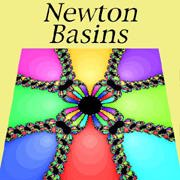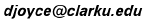# Newton Basin Generation

David E. JoyceClark University
August, 1994. Revised Jan., 1997

This form produces Newton Basins for polynomials. You can specify the polynomial by giving the number of roots, and for each root z = x + yi, its real part x, its imaginary part y, and its multiplicity m (usually 1). You may also specify the rectangle of the complex plane to display, the size of the image to be produced, and the maximum number of iterations of Newton's algorithm to be used for each pixel in the image.

Specify the polynomial by the number n (up to 6) of its roots:
(If you want more than 6 roots, use this alternate form.)

Next enter the n roots of the polynomial. For each root z = x + yi, enter x, y, and m, its multiplicity. (Only information for the first n roots is needed.)

 x1 = y1 = m1 = x2 = y2 = m2 = x3 = y3 = m3 = x4 = y4 = m4 = x5 = y5 = m5 = x6 = y6 = m6 =

Next specify the window of the complex plane to display. Give the coordinates of the center point z = x + yi and the "x-radius", that is, half the width of the window. The "y-radius" will by computed proportionally to the image rectange that you specify next.

Now specify the size (pixels by pixels) of the image you want to have.
Width = by Height =

Next, indicate the maximum number of iterations of Newton's method you would like for each pixel.
Maximum number of iterations:

Now, select whether you want each basin a single color, or each basin shaded according to how many iterations are needed to get close to the root.
Single color per basin

If you're all set, press the button to request the image.
(Note that it may take a while if you ask for a big image or a lot of iterations.)

Recently created images. Many interesting images are found by others.

Up to the Introduction.